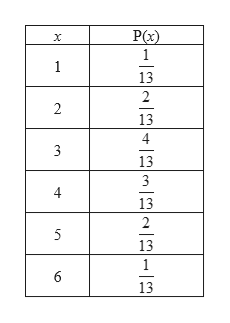Suppose you roll a die with probability of 1 being 1/13, 2 being 2/13, 3 being 4/13, 4 being 3/13, 5 being 2/13, and 6 being 1/13. What is the expected value of the up-face of the die?

Question
1. Suppose you roll a die with probability of 1 being 1/13, 2 being 2/13, 3 being 4/13, 4 being 3/13, 5 being 2/13, and 6 being 1/13. What is the expected value of the up-face of the die?
Step 1

The given values of up-face of the die and the corresp...help_outlineImage TranscriptionclosePx) 1 1 2 3 4 6 fullscreen

Want to see the full answer?

See Solution

Want to see this answer and more?

Our solutions are written by experts, many with advanced degrees, and available 24/7

See Solution
Tagged in

Other